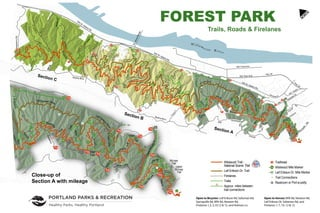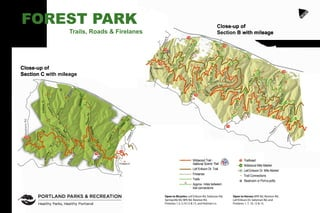Publicité1 sur 2
Publicité

### Forest Park trail map

1. FOREST PARK Trails, Roads & Firelanes To l i n d a T r a i l NW Yeon Ave. Newb erry Rd. S t . J o h n s B r i d g e NW Thompson R d NW Cornell NW Front Ave. NW St. Helens Rd. NW St. Helens Rd. NW St. Helens Rd. F i r e l a n e 1 2 F i r e l a n e 1 3 F i r e l a n e 1 5 B P A R d . N e w t o n R d . N e w t o n R d . F i r e l a n e 9 G e r m a n t o w n R d . F i r e l a n e 1 0 Firelane 7 Oil Line R d . G a s L i n e R d S a l t z m an R d . F i r e l a n e 5 S a l t z m a n Rd. F ire l a n e 3 F i r e l a ne 2 F i r e l a n e 1 Holman Lane NW 53rd Dr. C a n n o n T ra il S p r i n g vill e R d . W a t e r lin e T r a i l R i d g e T r a i l T r a i l H a r d e s t y T r a i l R i d g e M a p l e T r a i l M a p l e T r a i l F i r e l a n e 1 Chestnut Trail N a t u r e T r a i l T r a i l A ld e r D o g w o o d T r W i l d C h e r r y T r B i r c h T r L o w e r M c L e a y T r N W Rd. Cornell NW Thurman St. NW Nicolai St. Leif Erikson Drive Leif Eriks o n D r i v e W i l d woo d T r a i l W ildwo o d T r a i l Wil dwood T r a i l W i l d w o o d Trai l W i l d w o o d T r a i l W i l d w o o d T r S p r i n g ville Rd. C u mberland Upp e r McL e a y T r . Tunnel CumberlandRd. Aspen Trail NW 5 3 r d D r . W Burn s i d e R d . N W P i t t o c k D r . L e i f E rikson W ildwood Trail W i l l a m e t t e R i v e r L e i f Erikso n Hwy 30 Hwy 30 NW Vaughn N e w t o n R d . L i n n t on T r a i l T rillium Trail W i r egateTr. F i r e l a n e 4 Pittock Mansion 30 29 28 27 26 25 B P A R d . 24 23 22 21 20 19 18 17 16 15 14 13 12 11 10 9 8 7 6 5 4 3 11 10 9 8 7 6 5 4 3 2 1 0 N Trailhead Wildwood Mile Marker Leif Erikson Dr. Mile Marker Trail Connections Restroom or Port-a-potty 11 14 Wildwood Trail - National Scenic Trail Leif Erikson Dr. Trail Firelanes Trails Approx. miles between trail connections Open to Bicycles: Leif Erikson Rd, Saltzman Rd, Springville Rd, BPA Rd, Newton Rd, Firelanes 1,3, 5,10,12 & 15, and Holman Ln. Open to Horses: BPA Rd, Newton Rd, Leif Erikson Dr, Saltzman Rd, and Firelanes 1, 7, 10, 12 & 15. ne 2 F i r e l a n e 1 Holman Lane NW 53rd Dr. F i r e l a n e 1 C h e s t n u t T r a i l N a t u r e T r a i l A ld e r D o g w o o d T r W i l d C h e r r y T r B i r c h T r L o w e r M c L e a y T r d. Leif Erikson Driv e Leif Eriks o n D r i v e W i l d woo d T r a i l W ildwo o d T r a i l C u mberland Upp e r McL e a y T r . Tunnel Aspen Trail N W 5 3 r d D r . N W P i t t o c k D r . Pittock Mansion TH TH TH TH TH TH TH TH TH TH TH TH 13 12 11 10 9 8 7 6 5 4 3 4 3 2 1 0 Keil Trail McLeay Trail Upper McLeay Trail . 4 6 . 2 8 . 3 6 .3 8 . 2 3 .31 Close-up of Section A with mileage
2. To l i n d a T r a i l F i r e l a n e 9 G e r m a n t o w n R d . Firelane 7 Oil Line Rd G a s L i n e R d S a l t z m an R d . F i r e l a n e5 S a l t z m a n Rd. Fire l a n e 3 C a n n o n T ra il S p r i n g v i l l e R d . W a t e r li n e T r a i l R i d g e T r a i l T r a i l H a r d e s t y T r a i l R i d g e M a p l e T r a i l M a p l e T r a i l Wildwood T r a i l W i l d w o o d T r S p r i n g ville Rd. L e i f Eri k s on W i l d wood Trail L e i f Erikso n T rillium Trail W i r e gateTr F i r e l a n e 4 25 24 23 22 21 20 19 18 17 16 15 14 13 11 10 9 8 7 6 5 4 F i r e l a n e 2 M a p l e T r . .34 .48 .76 .17 .42 .69 .48 .74 .23 .93 .60 .78 .29 .32 .80 .62 .42 .26 .44 .20 Firelane 8 Close-up of Section B with mileage New berr y Rd. F i r e l a n e 1 2 F i r e l a n e 1 2 F i r e l a n e 1 3 F i r e l a n e 1 5 B P A R d . N e w t o n R d . N e w t o n R d . F i r e l a n e 9 G e r m a n t o w n R d . F i r e l a n e 1 0 W i l d w o o d Trai l W i l d w o o d T r a i l N e w t o n R d . L i n n ton T r a i l 30 29 28 27 26 25 B P A R d . B P A R d Firelane 8 N e w t on R d .78 .53 .90 .51 .37 .47 .45 .93 .51 .31 .38 .19 FOREST PARK Trails, Roads & Firelanes N Trailhead Wildwood Mile Marker Leif Erikson Dr. Mile Marker Trail Connections Restroom or Port-a-potty 11 14 Wildwood Trail - National Scenic Trail Leif Erikson Dr. Trail Firelanes Trails Approx. miles between trail connections Open to Bicycles: Leif Erikson Rd, Saltzman Rd, Springville Rd, BPA Rd, Newton Rd, Firelanes 1,3, 5,10,12 & 15, and Holman Ln. Open to Horses: BPA Rd, Newton Rd, Leif Erikson Dr, Saltzman Rd, and Firelanes 1, 7, 10, 12 & 15. Close-up of Section C with mileage
Publicité